Next: Shaping filters and the Up: Principles of norm and Previous: Principles of norm and

### Shaping filters and thenorm

This section illustrates some limitations of thenorm for the estimation of shaping filters. In figure, a simple 1-D problem is shown. On the top we have four events corresponding to one primary (on the left) and three multiples (on the right). Note that the primary has higher amplitude than the multiples. On the bottom we show a multiple model that exactly corresponds to the real multiples. Our goal is to estimate one shaping filterthat minimizes the objective function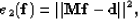(9)
where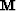is the matrix representing the convolution with the time series for the multiple model (Figureb) andthe time series for the data (Figurea). Now, if the filteris estimated with enough degrees of freedom (enough coefficients) to minimize equation (), we obtain for the estimated primaries, i.e.,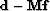, Figurea, and for the estimated multiples, i.e.,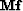, Figureb. The estimated primaries do not resemble the primary in Figurea. I show the corresponding shaping filter in Figure.datmul
Figure 1
(a) The data with one primary on the left, and three multiples on the right. (b) The multiple model that we want to adaptively subtract from (a).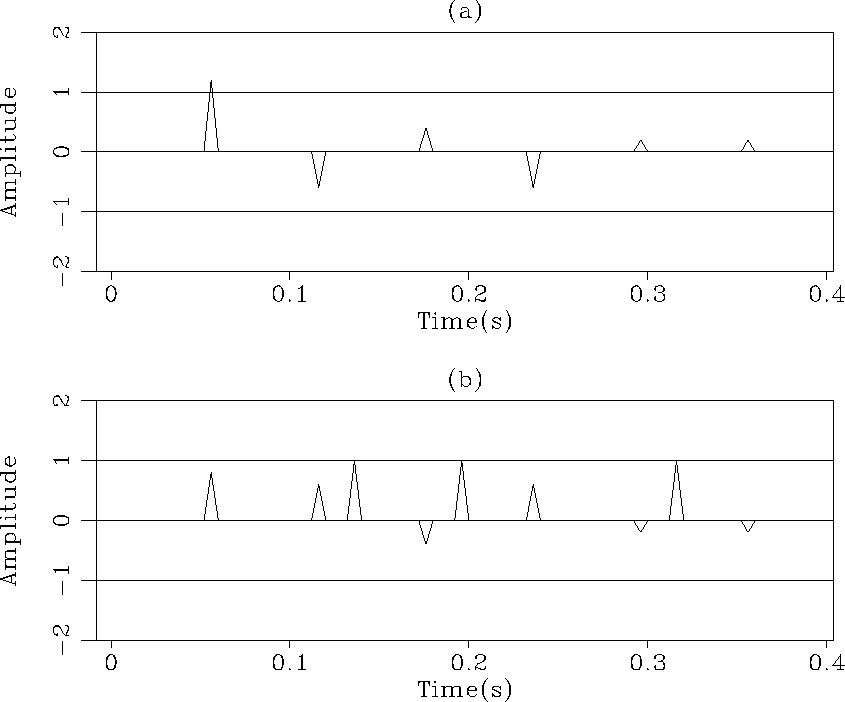1Dl2
Figure 2
(a) The signal estimated with thenorm. (b) The noise estimated with thenorm.This filter is not an unit spike at lag=0 as expected. The problem stems from the least-squares criterion which yields an estimated signal that has, by definition, minimum energy. In this 1-D case, the total energy in the estimated signal (Figurea) is e2=2.4, which is less than the total energy of the primary alone (e2=4). This is a fundamental problem if we use thenorm to estimate the shaping filter. In the next section, I show that the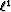norm should be used if amplitude differences exist between primaries and multiples.Next: Shaping filters and the Up: Principles of norm and Previous: Principles of norm and
Stanford Exploration Project
5/5/2005